## Example Questions

### Example Question #1 : How To Find The Volume Of A Prism

A rectangular prism has a width of 3 units, a length that is twice the width, and a height that is two-thirds the length. What is the volume of the prism?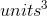Explanation:

Remember, the formula for the volume of a rectangular prism is width times height times length: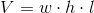Now, let's solve the word problem for each of these values. We know that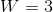. If length is double the width, then the length must be 6 units. If the height is two-thirds the length, then the height must be 4: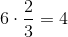Multiply all three values together to solve for the volume: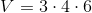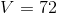The volume of the rectangular prism isunits cubed.

Tired of practice problems?

Try live online GRE Math prep today.